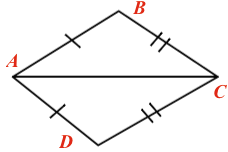### Home > CCG > Chapter 7 > Lesson 7.2.3 > Problem7-76

7-76.

For either part (a), (c), or (d) of problem 7-75, create a flowchart to prove your conclusion. Remember to start with the given information and include a reason or justification for each bubble in your flowchart.

Label each vertex. Start on the left with $A$, going clockwise label them $B$, $C$, and $D$.Given$\enclose{circle}[mathcolor="red"]{\color{black}{\; \\ \qquad \overline{AB} \cong \overline{AD} \qquad \\}}$ Given$\enclose{circle}[mathcolor="red"]{\color{black}{\; \\ \qquad \overline{BC} \cong \overline{CD} \qquad \\}}$ Reflexive$\enclose{circle}[mathcolor="red"]{\color{black}{\; \\ \qquad \overline{AC} \cong \overline{CA} \qquad \\}}$ $\huge \searrow$Bubble 1 has an arrow pointing down to bubble 4. $\huge \downarrow$Bubble 2 has an arrow pointing down to bubble 4. $\huge \swarrow$ Bubble 3 has an arrow pointing down to bubble 4. $\enclose{circle}[mathcolor="red"]{\color{black}{\; \\ \qquad \triangle ABC \cong \triangle ADC \qquad \\}}$$\quad \quad \quad \quad \quad \quad \quad SSS \cong$